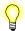# log(), logf(), logl()

Compute the natural logarithm of a number

## Synopsis:

```#include <math.h>

double log( double x );

float logf( float x );

long double logl( long double x );```

## Arguments:

x
The number that you want to compute the natural log of.

## Library:

libm

Use the -l m option to qcc to link against this library.

## Description:

The log() and logf() functions compute the natural logarithm (base e) of x:

loge x

A domain error occurs if x is negative. A range error occurs if x is zero.

## Returns:

The natural logarithm of x. For finite values of x less than zero, these functions return NaN. The return value when x is -Inf is NaN.If an error occurs, these functions return 0, but this is also a valid mathematical result. If you want to check for errors, set errno to 0, call the function, and then check errno again. These functions don't change errno if no errors occurred.

## Examples:

```#include <stdio.h>
#include <stdlib.h>
#include <math.h>

int main( void )
{
printf( "%f\n", log(.5) );

return EXIT_SUCCESS;
}```

produces the output:

`-0.693147`

## Classification:

Safety:
Cancellation point No
Interrupt handler No
Signal handler No